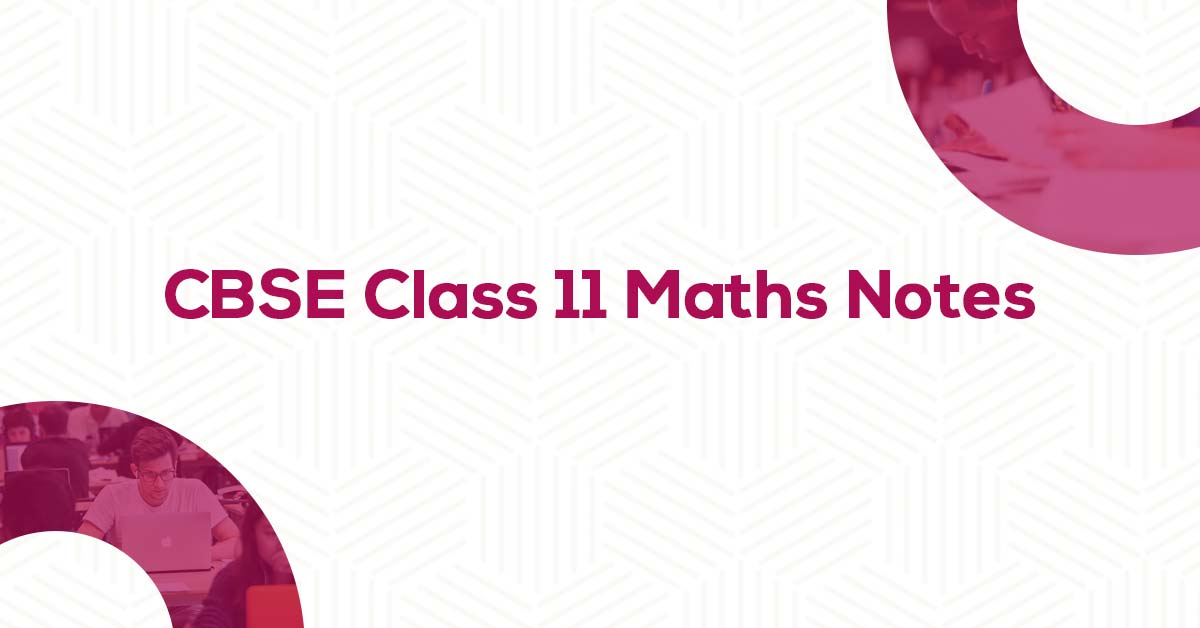Class 11 Maths Formulae & Notes PDF | Vidyakul
×

# CBSE Class 11 Maths Formulae & Notes

##Vidyakul brings CBSE Class 11 Maths Formulae, cumulated by our panel of highly qualified teachers, strictly according to the NCERT Syllabus to ease out the preparation and revision process of the students for the exams. These pdf not only include the list of formulae but offer students with the summary of the chapters, important points to remember, detailed explanation of important concepts and derivations of formulae for better comprehension and retaining of the chapters. Thus, these Chapter-wise CBSE Class 11 Maths Formulae are prepared to ensure maximum preparation and good marks in the examination.

## Chapter-wise CBSE Class 11 Maths Formulae:

Class 11 Maths is considered to be a challenging subject for many students. Most of the time, students develop a phobia for Mathematics that mostly take an ugly turn in their final results. So continuous efforts and rigorous practice are required by the students to score maximum marks in the examinations.

### Related Class 11 Notes Chapter-wise:

• Class 11 Physics Notes
• Class 11 Biology Notes
• Class 11 Chemistry Notes

• The CBSE Class 11 Maths Formulae are cumulated by our panel of highly experienced teachers after analyzing the past 10 years of examination papers and material so that no important formula is left behind. This utmost precision in preparing these Formulae for the students ultimately leads to maximum marks in the examination.

Vidyakul understands the difficulties faced by the students in Class 11 while facing Maths, thus, we bring them a solution to lower down the pressure and increase their self-confidence. Free Download of CBSE Class 11 Maths Formulae pdf would help students in effective preparation and revision for the examinations.

• NCERT Solutions Class 11 Maths
• NCERT Solutions Class 11 Physics
• NCERT Solutions Class 11 Chemistry
• Class 12 Maths Formulae
• Class 12 Physics Notes
• Class 12 Biology Notes
• Class 12 Chemistry Notes
• NCERT Solutions Class 12 Maths
• NCERT Solutions Class 12 Physics
• NCERT Solutions Class 12 Chemistry
•